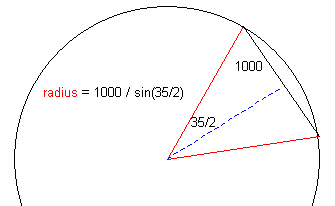SEARCH HOMEMath Central Quandaries & QueriesQuestion from Cindy, a student: There is a railroad curve with a chord length of 2000 ft. and a central angle of 35 degrees. What is the radius and arc length of the circular arc?Hi Cindy.First find the radius.  If you draw the diagram with radii you will realize you have an isosceles triangle whose base length and angles you know.  Thus you can divide it into two congruent right triangles and calculate the radius using sine.

Once you have the radius, the arc length is easy.  A full circle is 360 degrees but your arc is 35 degrees of that.  So you have 35/360 of the circumference of a circle whose radius you know.

Cheers,
Stephen La Rocque.Math Central is supported by the University of Regina and The Pacific Institute for the Mathematical Sciences.